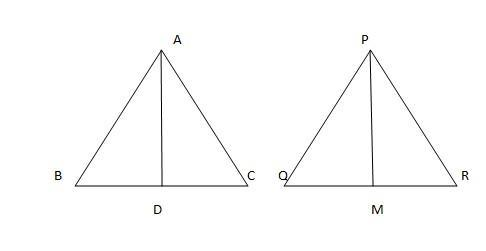Courses
Courses for Kids
Free study material
Free LIVE classes
More# If AD and PM are medians of triangles ABC and PQR , respectively where $\vartriangle ABC \sim \vartriangle PQR$ , prove that $\dfrac{{AB}}{{PQ}} = \dfrac{{AD}}{{PM}}$Verified
327.3k+ views
Hint: Let’s make use of the property of congruence of triangles & analyse the sides of triangles by medians to approach the solution.Given that $\vartriangle ABC \sim \vartriangle PQR$

So, from the property of congruence triangles, we can write
$\dfrac{{AB}}{{PQ}} = \dfrac{{BC}}{{QR}} = \dfrac{{AC}}{{PR}}$ (Corresponding sides of congruent triangles)

Also, $\angle A = \angle P,\angle B = \angle Q,\angle C = \angle R$ (Corresponding angles of congruent triangles)

Given that AD and PM are medians of the triangle ABC and PQR respectively

If a median is drawn from a vertex to a side, then it divides the side equally
So, from this we can write BD=CD$\Rightarrow BC = 2BD$
QM=MR$\Rightarrow$QR=2QM

Hence, from this we can write
$\dfrac{{AB}}{{PQ}} = \dfrac{{2BD}}{{2QM}} = \dfrac{{AC}}{{PR}}$
$\Rightarrow \dfrac{{AB}}{{PQ}} = \dfrac{{BD}}{{QM}} = \dfrac{{AC}}{{PR}}$
So, now we can write in $\vartriangle ABD$ and $\vartriangle PQM$
$\dfrac{{AB}}{{PQ}} = \dfrac{{BD}}{{QM}}$
and $\angle B = \angle Q$

And so , from SAS similarity, we can write
$\vartriangle ABD \sim \vartriangle PQM$
$\therefore \dfrac{{AB}}{{PQ}} = \dfrac{{AD}}{{PM}}$ (Congruent sides of congruent triangles)

So, this is what we had to prove, hence the result is proved.

Note: When solving these types of problems first prove the congruence of the triangles and from this find out the ratio of the corresponding sides in accordance to the RHS which has to be proved.
Last updated date: 03rd Jun 2023
Total views: 327.3k
Views today: 4.83k